• pandas中DataFrame的“索引列”，删除pandas中的列关于DataFrame中的“索引列删除列方法一：del df['index']方法二：df.drop(['index'],axis = 1)删除索引列” 关于DataFrame中的“索引列” 在DataFrame中的索引...


pandas中DataFrame的“索引列”，删除pandas中的列
关于DataFrame中的“索引列”删除列方法一：del df['index']方法二：df.drop(['index'],axis = 1)
删除“索引列”

关于DataFrame中的“索引列”
在DataFrame中的索引都在最左边的一列中，暂且叫它“索引列”，但其实它不是一个列，所以适用于删除列的命令都不适合它
删除列
方法一：del df[‘index’]
score=pd.read_csv('./dietplan.txt',sep='\t',index_col='Participant')
scorescore=pd.read_csv('./dietplan.txt',sep='\t',index_col='Participant')
del score['Diet-Plan1']
score但如果想用del删除设定为索引的那一列，就会报错：
score=pd.read_csv('./dietplan.txt',sep='\t',index_col='Participant')
del score['Participant']
score方法二：df.drop([‘index’],axis = 1)
score=pd.read_csv('./dietplan.txt',sep='\t',index_col='Participant')
score=score.drop(['Diet-Plan1'],axis=1)
score如果想用drop方法删除设定为索引的那一列，也会报错：
score=pd.read_csv('./dietplan.txt',sep='\t',index_col='Participant')
score=score.drop(['Participant'],axis=1)
score删除“索引列”
如果DataFrame中的“索引列”是一个有实际意义的属性，例如：这个表中的“Rank”是索引，但是同时它也代表了这些学校的2018的QS排名。
如果需要删除这一列，那么则需要去除这一列的索引属性，就是不把这一列设为索引。
qs = pd.read_excel('./2018-QS-World-University-Rankings-Top200.xlsx',index=False)

然后再用上面的两种方法进行删除
展开全文python pandas
• DataFrame数据集索引转换和重命名 DataFrame数据结构类型我们常见的excel表格一样，直观简单利于理解。 该数据集有行和列及索引的...1.查看数据索引列 col_name = bin_df.index.name print(‘col_name:’,col_name...
DataFrame数据集索引转换和重命名

DataFrame数据结构类型我们常见的excel表格一样，直观简单利于理解。 该数据集有行和列及索引的概念。 我们在数据操作中常常需要进行的对数据集进行分组统计之类。这时就很涉及到数据集改变之后数据索引也可能随之改变。

1.查看数据索引列

col_name = bin_df.index.name print(‘col_name:’,col_name) print(bin_df) 注：bin_df是数据集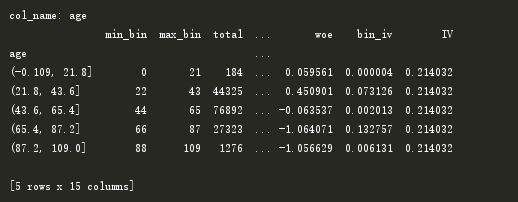发现索引列是我们关心的age列，需要将其转换成特征列，即:reset_index()

bin_df = bin_df.reset_index() col_name = bin_df.index.name print(‘col_name:’,col_name) print(bin_df)

结果如下：发现age已经转换成特征列。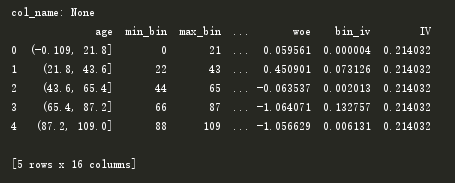2.给数据集重新命名新的索引列

print(‘col_name:’, bin_df.index.name) bin_df.index.name = ‘num’ print(bin_df) print(‘col_name:’, bin_df.index.name)

结果如下：修改索引成功！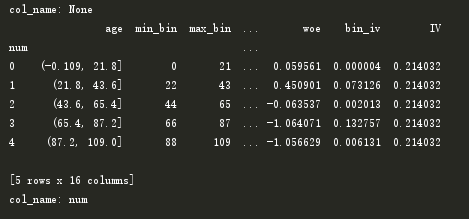3.指定多个列作为多级索引

bin_df = bin_df.set_index([‘age’,‘woe’]) print(bin_df) print(‘col_name:’, bin_df.index.names)

结果如下：需要注意的是多层索引名的输出时用的是bin_df.index.names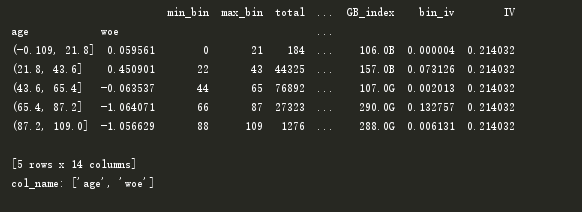同时保留作为索引的列

bin_df = bin_df.set_index([‘age’,‘woe’],drop=False) print(bin_df) print(‘col_name:’, bin_df.index.names)

注：drop= False，在列中保留了作为索引的列，等于True时直接删除。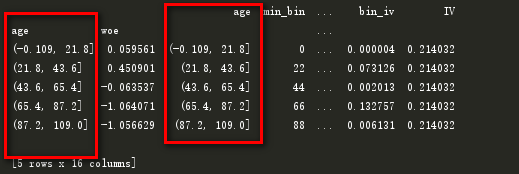4. 使用rename方法：

DataFrame.rename（mapper = None，index = None，columns = None，axis = None，copy = True，inplace = False，level = None ） 参数介绍： mapper，index，columns：可以任选其一使用，可以是将index和columns结合使用。index和column直接传入mapper或者字典的形式。 axis：int或str，与mapper配合使用。可以是轴名称（‘index’，‘columns’）或数字（0,1）。默认为’index’。 copy：boolean，默认为True，是否复制基础数据。 inplace：布尔值，默认为False，是否返回新的DataFrame。如果为True，则忽略复制值。

展开全文• ## Dataframe列索引操作

千次阅读 2019-06-01 23:08:01
1.在df的索引’Salary’左侧插入索引’low-salsary’并赋值 col_name = data.columns.tolist() col_name.insert(col_name.index('Salary'),'low_salary') df = df.reindex(columns=col_name) df['low_salary'] =...
1.在df的索引’Salary’左侧插入索引’low-salsary’并赋值
    col_name = data.columns.tolist()
col_name.insert(col_name.index('Salary'),'low_salary')
df = df.reindex(columns=col_name)
df['low_salary'] = low_list

2.将索引名’Salary’:改为’high_salary’并赋值
df.rename(columns={'Salary': 'high_salary'},inplace=True)
df['high_salary'] = high_list

3.自定义删除函数
def drop_eduyc(df,columns): # 利用drop方法将colums='educationBackground'中含有'招'字的行删除
cols = [x for i, x in enumerate(df.index) if u'招' in df.loc[i, columns]]
# 利用enumerate对columns进行遍历，将colums='educationBackground'中含有'招'字的educationBackground放入cols中
df.drop(cols, axis=0,inplace=True)#删除含有‘招’字的行
drop_eduyc(data, 'educationBackground')#调用函数

展开全文• ## DataFrame多重索引

千次阅读 2019-09-03 23:19:36
DataFrame多重索引，分组计算
1、多重索引
s = pd.Series(np.random.randint(-10,10,7),
index=[['a','a','b','b','c','c','c'],[0,1,0,1,0,1,2]])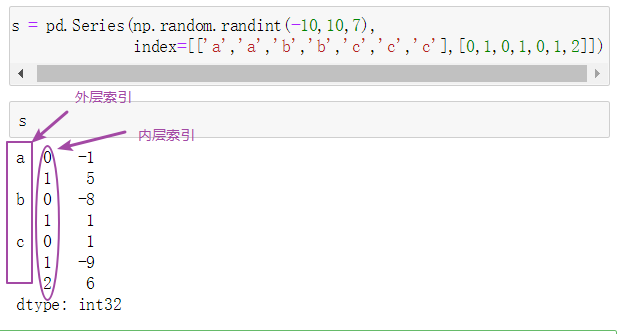上图中codes（有些版本中是labels）对应着levels的下标，codes中的两个列表一一对应 例如：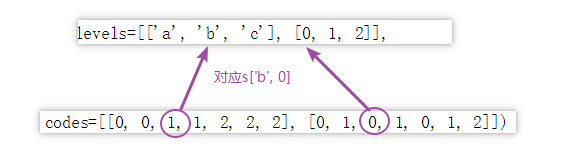多重索引取值
1、取外层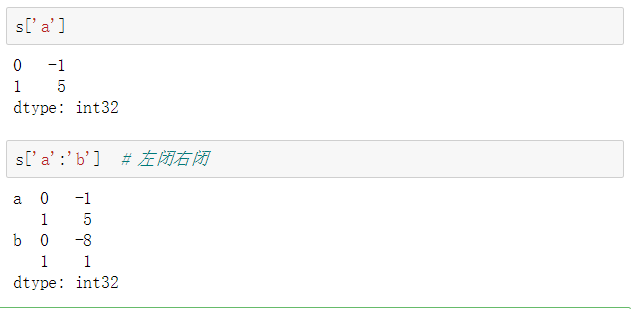2、取内层 s[ :, 0]表示取每一个外层索引对应的内层索引为0的数，即所有内层索引为0的数，共有三个数内层索引为0转换内外层索引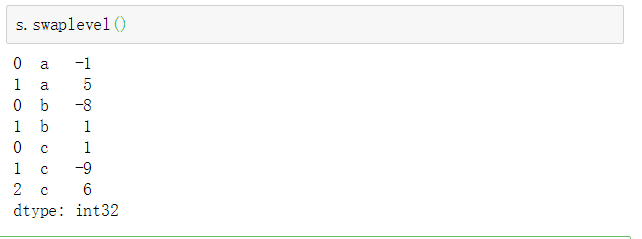dataFrame多重索引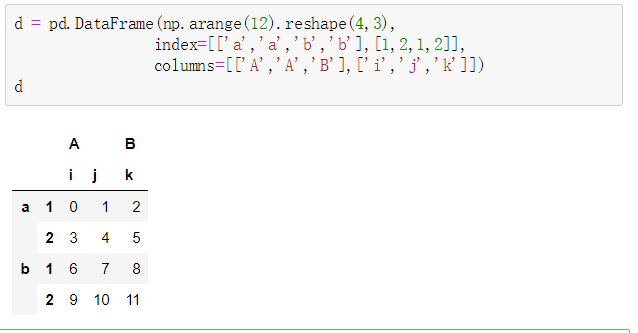取值2、Series和DataFrame的转换
1、series转DataFrame 索引不完整的用nan填充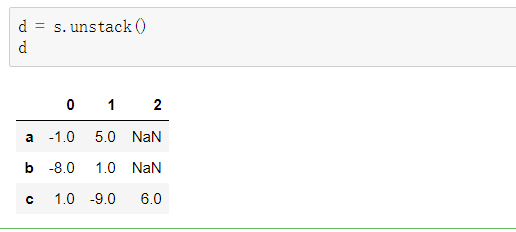2、dataframe转series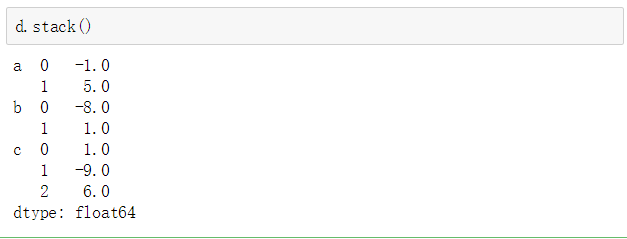3、DataFrame重置索引
先取电影数据的前50条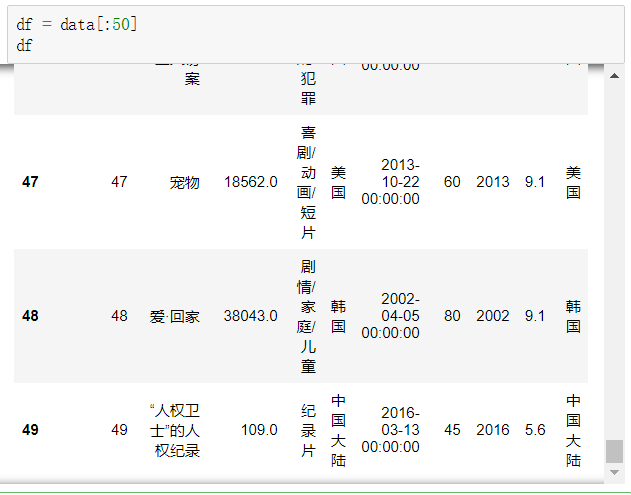将产地和年代设置为索引，产地为外层索引，年代为内层索引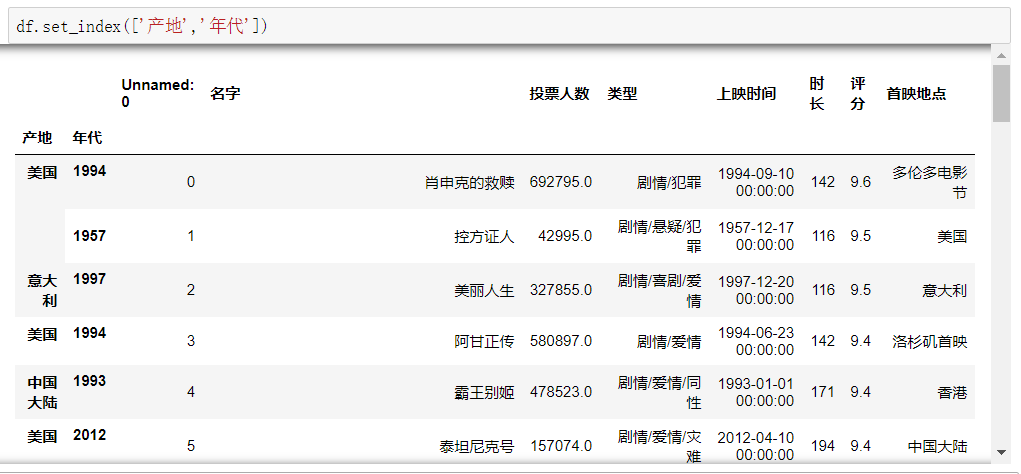通过外层索引获取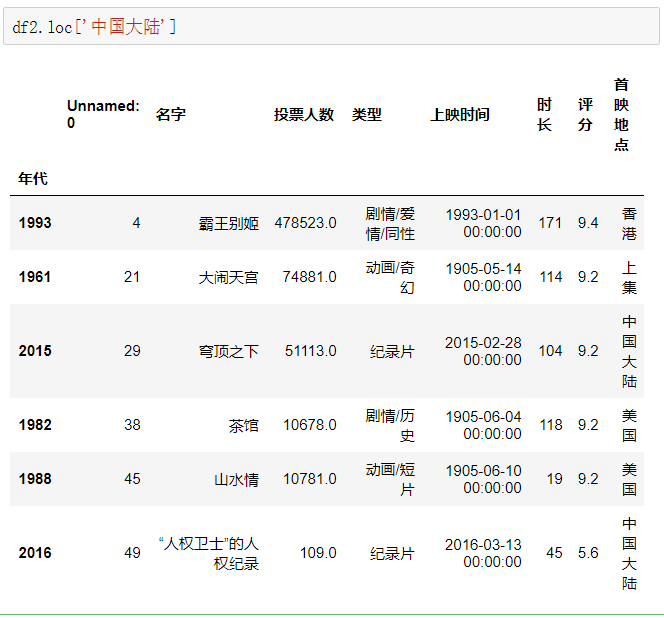转换内外索引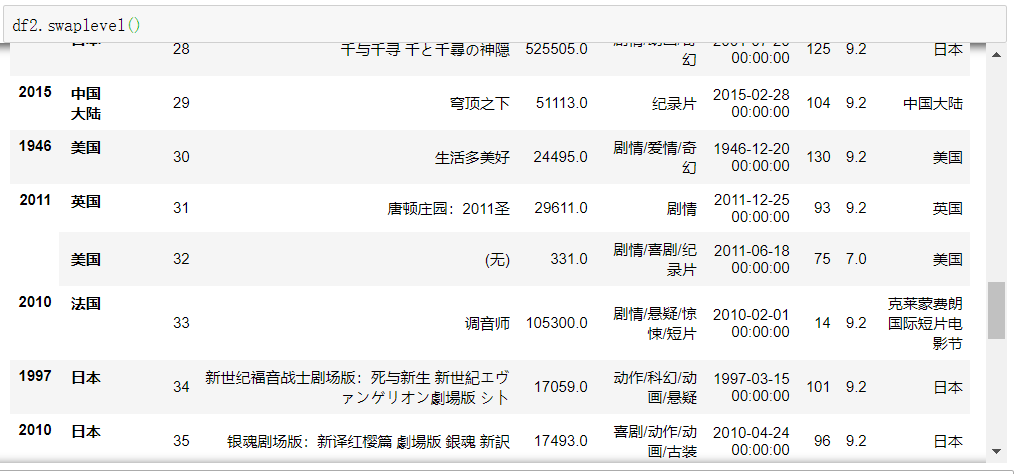取消内外层索引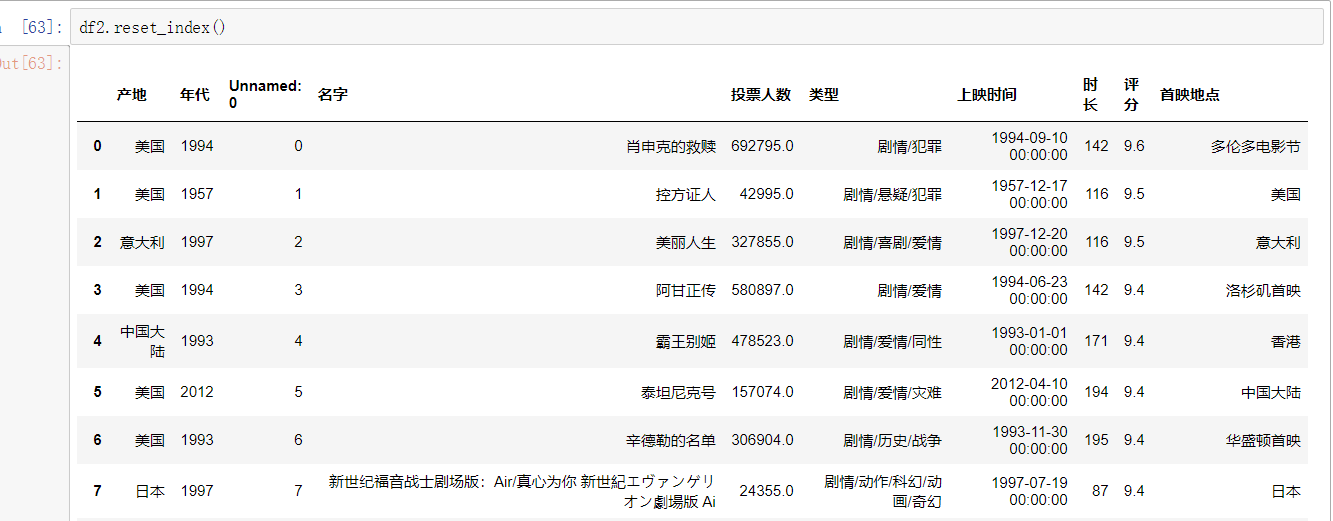展开全文pandas
• 首先讲讲根据行列索引的查询 import pandas as pd import numpy as np df = pd.DataFrame(np.random.randint(50, 100, size=(4, 4)), columns=pd.MultiIndex.from_product( [['math', 'physics'], ['term1', '...python pandas
• 用布尔索引的方法删除DataFrame中的行或 对于pandas DataFrame对象通常用“对象名.drop()”方法删去行或，但drop操作必须先知道想要删除的列名和索引。 本Markdown编辑器使用StackEdit修改而来，用它写博客，...
• ## DataFrame的索引

千次阅读 2018-05-30 10:55:44
DataFrame可以同list或者ndarray一样，根据下标索引，如tset，同时，由于index和columns属性，有一些其他的索引方法。一个简单的DataFrame如下test = pd.DataFrame([[1,2,3,4],[5,6,7,8]],index = ['a','b'],...
• 我们常常需要将DataFrame对象中的某或某几列作为索引，或者将索引转化为对象的。pandas提供了set_index()/reset_index() 来供我们使用。 一、转化为索引 df1=pd.DataFrame({'X':range(5),'Y':rang...pandas python
• DataFrame的行列索引命名 import numpy as np import pandas as pd df=pd.read_excel('C:\\****.xlsx') #####给行列索引命名 df.index.names=['行'] df.columns.names=[''] print(df.head()) #######输出行...python 数据分析
• 在Pandas中，自定义索引后，有时候又需要恢复默认索引。 import pandas as pd import numpy as np df = pd.DataFrame(np.arange(20).reshape(5,4),columns=['a','b','c','d']) df a b c d 0...数据分析 python
• 通过isnull我们发现某有几个空值，要把该空值所在行删除怎么操作？用dropna()会删除所有有空值的行，请看下面实例。 该函数主要用于滤除缺失数据。 如果是Series,则返回一个仅含非空数据和索引值的Series，默认...
• 一、DataFrame索引操作1.1 修改行索引名1.2 修改列索引名1.3 设置某一列为新的索引二、DataFrame的拼接2.1 append方法2.2 concat方法三、DataFrame的关联3.1 merge方法3.2 join方法 一、DataFrame索引操作 import ...python
• ## 从DataFrame中删除列

千次阅读 2021-03-16 09:34:13
在操作数据的时候，DataFrame对象中删除一个或多个是常见的操作，并且实现方法较多，然而这中间有很多细节值得关注。 首先，一般被认为是“正确”的方法，是使用DataFrame的drop方法，之所以这种方法被认为是标准...python 机器学习 数据挖掘
• DataFrame的行名称为日期 date=pd.date_range（start='20210201',periods=5,freq='B'） dataframe索引名显示为2021-02-01到2021-02-05 删除列时data.drop（['2021-02-04]',axis=1） 报错KeyError:['2021-02-04']...python
• When deleting a column in a DataFrame I use: 在删除DataFrame中的时，我使用： del df['column_name'] And thipython pandas
• 一，设置DataFrame索引值 以及 时间索引如何构造 1，设置DataFrame索引值 import numpy as np import pandas as pd day_data = np.random.normal(0,1,(500,507)) # 将数据变成dataframe格式 day_data1 = pd....python 数据分析
• 在Python数据处理中如果实现excel透视表中的功能，则多重索引问题就比较突出了。近来为实现Python自动邮件，需要对数据进行透视表功能，遇到多重索引和多表头问题，下面总结下用法。 一、多重索引 常规的单索引这里...
• 文章目录2、DataFrame1）DataFrame的创建DataFrame属性：values、columns、index、shape、ndim、dtypes2）DataFrame索引(1) 对进行索引错误演示，取行的索引(2) 对行进行索引(3) 对元素索引的方法使用行索loc引...数据结构 python numpy java 机器学习
• .reindex()能够改变或重排Series和DataFrame索引 .reindex(index=None, columns=None,…)的参数 参数 说明 index, columns 新的行列自定义索引 fill_value 重新索引中，用于填充缺失位置的值 ...
• Pandas数据处理 ...当在两个Series或DataFrame对象上进行二元计算时，Pandas会在计算过程中对齐两个对象的索引。 Series索引对齐 A=pd.Series([2,4,6],index=[0,1,2]) B=pd.Series([1,3,5],index=...
• 如何删除python pandas.DataFrame 的多重index遗忘是小编们不可更改的宿命，所有的一切都像是没有对齐的图纸，从前的一切回不到过去，就这样慢慢延伸，一点一点的错开来，也许，错开了的东西，小编们真的应该遗忘了......